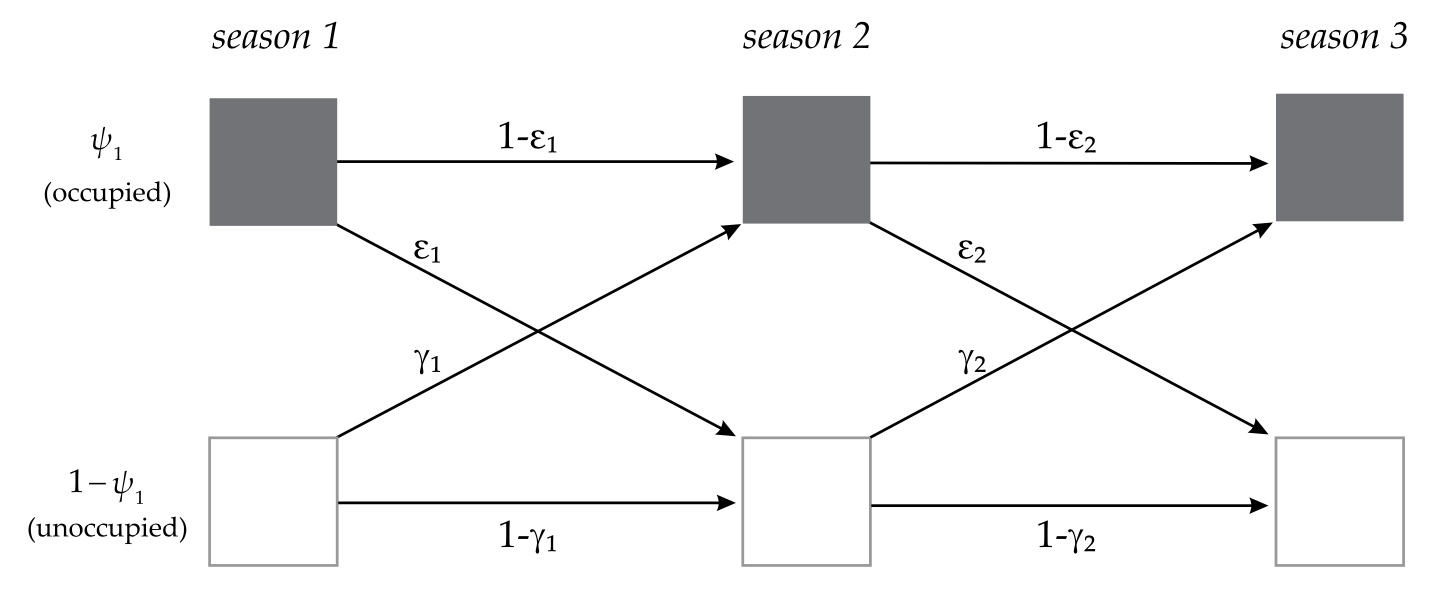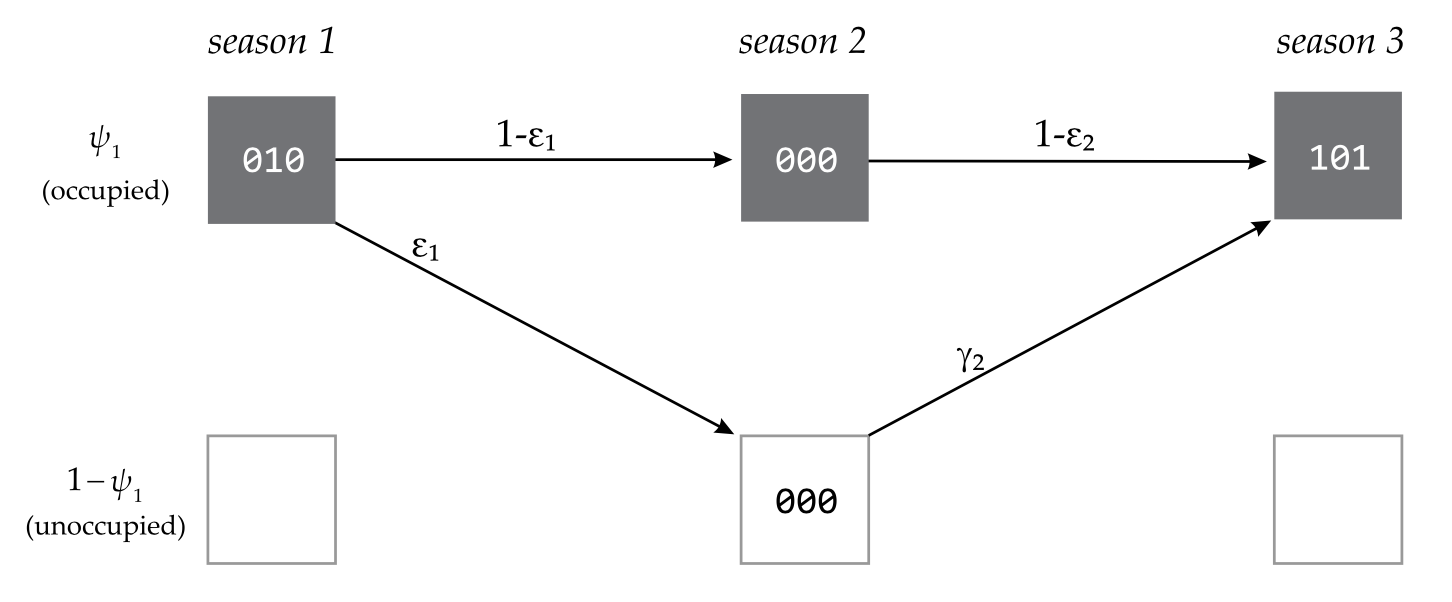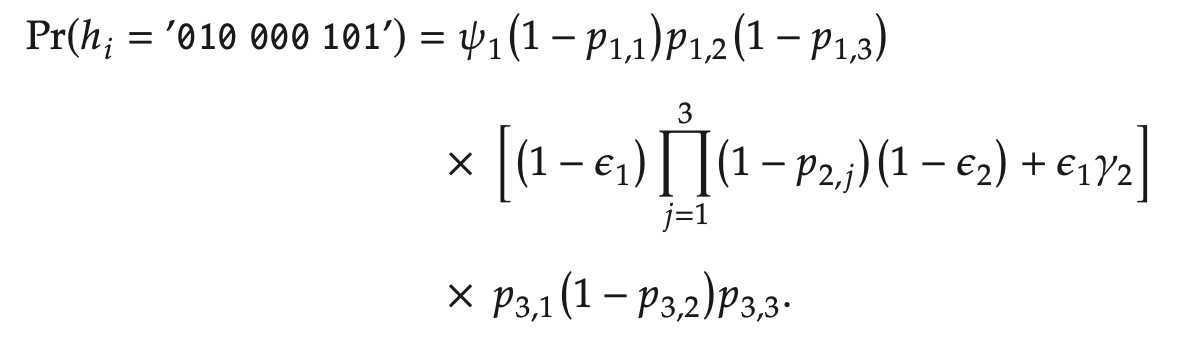Core Concept

Section 21.6 of Ch. 21 of CW, which was written by Brian D. Gerber, Brittany Mosher, Daniel Martin, Larissa Bailey, and Thierry Chambert, opens with a nice summary that is quoted below on the concept of dynamic, or multi-season, occupancy models.

A natural extension of the basic occupancy model (single species, single season) is to link several ’seasons’ together to investigate site-level dynamics. This allows researchers to focus on the processes that govern occurrence patterns, such as how and why occupied sites become extirpated (or conversely, persist) or how and why unoccupied sites become colonized.

With multi-season occupancy models, you can obtain time-specific rates of the following while accounting for imperfect detection.

1. Occupancy in each year and so occupancy dynamics through time
2. Rates of extirpation and colonization
• think about how would these be affected if ignore p and p<1)
• can model using covariats,
• e.g., model rates as functions of patch size, isolation, and/or connectivity
• e.g., model rates as function of annual features such as weather and/or management actions
1. With rates estimated, you can evaluate equilibrium occupancy rates

With the multi-season approach, you use multiple surveys within each primary occasion, e.g., year, and work across multiple primary occasions. The model makes the assumption that occupancy status does not change within a primary occasion but might change across primary occasions through colonization and extinction events. Thus, the approach is quite similar in concept to the robust design model for mark-recapture data on individually marked animals.

Parameters

The parameters are as follows.

• $$\psi_t$$: occupancy rate by year,

• $$\lambda_t = \frac{\psi_{t+1}}{\psi_{t}}$$: rate of change in occupancy,

• $$\epsilon_t$$: probability that a site that was occupied in primary occasion $$t$$ is unoccupied in season $$t + 1$$, i.e., extirpation rate,

• $$\gamma_t$$: probability that a site that was unoccupied in primary occasion $$t$$ is occupied in season $$t + 1$$, i.e., colonization rate.

As you’ll see in this week’s homework, there are several alternative parameterizations of the dynamic occupancy model that can be implemented in program MARK. We’ll begin by focusing on a version that focuses on occupancy in the first primary occasion (hereafter, season), $$\psi_1$$, and time-specific rates of extirpation and colonization. As illustrated on page 21-25 of Ch. 21 of CW and shown below, a site’s occupancy status can change from its initial status ($$\psi_1$$) because (a) the individuals at occupied sites can persist or be extirpated ($$\epsilon_t$$) and (b) unoccupied sites can be colonized ($$\gamma_t$$) or not.Occupancy status after the first season can be derived from initial occupancy and rates of extirpation and colonization as follows.

$\psi_{t+1} = \psi_t (1-\epsilon_t) + (1-\psi_t) \gamma_t$

Probability Statements for Encounter Histories

As before with single-season occupancy (and the previously covered mark-recapture models), we can write probability statements for each encounter history based on the model structure and its parameters. Within any season in which the species was detected at least once, the occupancy status is known as the model being used here assumes no false positives. Thus, any 0’s recorded on other secondary occasions within that season for that site are failed detections ($$1-p_t$$). As shown below in the illustration from page 21-26 of Ch 21 of CW, the probability statements consider all of the possible ways that the history might have occurred.In the case illustrated, in the second season, the site, which was known to have been occupied in the first season, could have remained occupied but each of the 3 attempts to detect the species failed. Alternatively, the site could have been extirpated. Because the species was observed in the third season, that information is also included in the probability statement. The probability statement from page 21-26 of Ch 21 of CW nicely lays out the probability of the history for each of the 3 seasons on separate lines and connects them with multiplication, the probability of season 1’s history and the probability of season 2’s history and the probability of season 3’s history.Notice in the probability statement that both possibilities are represented for what might have happened in the second season when the species was not detected (see line 2). The species could have persisted and gone undetected in season 2, in which case it is known to have also persisted into season 3. Or (represented by a ‘+’ sign in the second line of the probability statement above), the species could have gone extinct and then the site was colonized between seasons 2 and 3. For the second possibility (extinction followed by colonization), detection doesn’t enter in because the species was not there to be detected.

Working with the Output

Equilibrium Occupancy

As you’ll see in the homework, there are a few ways to evaluate whether the population is at, or headed for, an equilibrium occupancy rate, $$\psi_{eq}$$. One possibility is that $$\epsilon$$ and $$\gamma$$ are constant. If so, $$\psi_t$$ will eventually settle on a fixed value. For example, if $$\psi_1 = 0.7$$ and $$\epsilon = 0.2$$ $$\gamma = 0.6$$, let’s work out how $$psi_t$$ will change over time. Remember from above that $$\psi_{t+1}$$ can be obtained as follows.

$\psi_{t+1} = \psi_t (1-\epsilon_t) + (1-\psi_t) \gamma_t$

If you work out the values for years 2 through 10, you’ll find that $$\psi_2 = 0.744$$, $$\psi_3 = 0.7488$$, …, $$\psi_9 = 0.7499999$$, and $$\psi_{10} = 0.75$$. From that point, $$\psi_t$$ will not change: $$0.75 \cdot (1 - 0.2) + (1 - 0.75) \cdot 0.6 = 0.6 + 0.15 = 0.75$$. That is, even though colonization and extirpation still go on, they are off-setting such that the overall proportion of sites that are occupied remains unchanged or is at equilibrium.

It turns out that for this scenario, you can calculate $$\psi_{eq}$$ from $$\epsilon$$ and $$\gamma$$. In particular, $$\psi_{eq} = \frac{\gamma}{(\gamma + \epsilon)}$$. For the example above, $$\psi_{eq} = \frac{0.6}{(0.6+0.2)} = \frac{0.6}{0.8} = \frac{3}{4}=0.75$$.

You might have seen this relationship before if you’ve studied the classic metapopulation model of Levins (1970). It’s important to realize that for this equation for $$\psi_{eq}$$ to hold, it is assumed that colonizers are always available (colonization rate does not depend on the current occupancy pattern), i.e., there is a large outside (mainland) source. This, of course, may or may not be true for the situation at hand. In particular, you would want to consider if it is reasonable to assume that there is a large, outside source of colonizers and that $$\epsilon$$ and $$\gamma$$ are likely to remain constant over time.

In the homework, you’ll also explore models that constrain $$\epsilon = (1-\gamma)$$. If this model is appropriate for the study system, the equation $$\psi_{eq} = \frac{\gamma}{(\gamma + \epsilon)}$$ still holds. But, equilibrium will be attained immediately. For example, if $$\psi_1 = 0.2$$, $$\gamma = 0.6$$, and $$\epsilon = 1-\gamma$$ (or $$0.4$$), then $$\psi_2 = 0.2 \cdot (1-0.4) + (1 - 0.2) \cdot 0.6 = 0.12 + 0.48 = 0.6$$, which is $$\psi_{eq}$$ because $$\psi_{eq} = \frac{\gamma}{(\gamma + \epsilon)} = \frac{0.6}{(0.6+0.4)} = \frac{0.6}{1} = 0.6$$.

Another, perhaps simpler, method for evaluating equilibrium is to evaluate the support provided by the data for a model in which both $$\epsilon$$ and $$\gamma$$ are zero. Clearly, under such a scenario, $$\psi_t$$ won’t change as no colonization or extirpation events occur.

Derived parameters

From estimates of $$\psi_1$$, $$\epsilon_t$$, and $$\gamma_t$$, you can derive estimates of $$\psi_t$$ and $$\lambda_t$$, the change in occupancy rate through time. As noted on page 21-32 of Ch 21 of CW, you can also work with the ratio of the odds of occupancy at time $$t$$ versus at time $$t + 1$$, which can be of interest in some cases.

Derived $$\psi_t$$

For many studies, you will likely have interest in using temporal and spatial covariates and be interested in how $$\psi_t$$ is predicted to change under different covariate conditions. Doing so in the face of imperfect detection and while expressing uncertainty, i.e., $$\widehat{SE}(\hat\psi_t)$$, can be of great interest in some situations.

Alternative Software for Occupancy Modeling

There are other platforms for implementing occupancy models. For example, Program PRESENCE as well as R packages RPresence and unmarked provide alternatives. The software package GenPres offers the ability to conduct simulation studies that can be helpful in designing studies.

Study Design

If you get involved in occupancy modeling, it is important to be aware of, and take advantage of, the fact that there is a fair bit of literature dedicated to evaluating the efficacy of various study designs. For example, what are the tradeoffs between visiting more sites with fewer visits per site versus visiting fewer sites more times.